### Patterns of Patterns

As a young math student I knew tons of formulas and how to use them, but when it came to counting and generalizing sequences of numbers I often had to resort to brute force, or at least guess-and-check. It frustrated me that I knew there should be an easier way to generalize number patterns, but because I could usually get the right answer (patience and accuracy were on my side, thankfully), I didn't force myself to understand patterns more deeply.

For my first three years of teaching I used the original CPM series, and in their Algebra (Math 1) text they presented students with three key number sequences: the square numbers, the rectangular numbers, and the triangular numbers. These sequences appear so frequently that knowing and understanding their generalizations can be helpful when the sequences appear explicitly or when they are embedded in the foundation of another pattern.

The square number pattern, shown below, is the simplest of the three patterns, although students who are struggling to move beyond a recursive view of the pattern are likely to describe the sequence as "adding the next consecutive odd number." Because of this, the square numbers become a great example for students to see the importance of moving beyond recursive descriptions of patterns and towards expressions that yield the number of tiles for any figure.

The rectangular numbers are the next sequence in the progression of patterns. Instead of the expression(or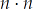) as with square numbers, the rectangular numbers usefor one dimension.
The triangular numbers look like a new pattern:

But in fact, the triangular numbers can be seen as half of each rectangular number. This means we can modify the rectangular number expression and represent triangular numbers using.
There are real-life representations of the triangular numbers, for sure (bowling pins, stacks of cans or boxes, etc.), but the real mathematical power behind leading students through this progression is that they see two examples of how to modify a previous pattern and generalization to get a new pattern and generalization.

Our class was presented with a problem known as the "Skeleton Tower," borrowed from http://www.wcer.wisc.edu/archive/nise/Publications/Briefs/Vol_2_No_1/. I've attempted to re-illustrate the tower in the figure below:
Suddenly our figures jump from 2-dimensional to 3-dimensional, but in doing so we've opened up the strategies students might use to generalize the pattern. If a student looks at how the construction of the tower progresses from figure to figure, he or she may think of the tower as a sum of horizontal layers. If a student were only given the table, or the sequence 1, 6, 15, 28,..., he or she would most likely see the mathematics of the sequence the same way.

On the other hand, if a student were familiar with the power of the square, rectangular, and triangular numbers, they may spatially reason the tower to be a single column of blocks of height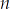, surrounded by four "wings," each in the pattern of the triangular numbers. Because the height of each triangular wing is, not, an adjustment to the triangular number expression must be made, giving us a new expression ofto describe each of the tower's wings. All together, we can express the number of blocks in a skeleton tower of height as follows:

If a student were to rewrite this expression as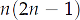, they might visualize the tower as rearranged with two opposite wings removed and each stacked on top of the remaining wings, making a rectangular shape with heightand width. Remember, the triangular wings were undersized to begin with; the only reason the width is not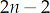is because the center column remains in the middle. Here is the pattern "flattened," which hints at solutions using both the overall dimensions (as just discussed) or using the rectangular numbers plus a center column:

The progression through the square, rectangular, and triangular numbers certainly makes the skeleton tower more approachable. But what if we build a tower that is more complex? What if our three fundamental number sequences are more hidden, and spatial "flattening" strategies are less apparent? Below is one such possible tower, an extension of the skeleton tower.

Unfortunately, after several hours of trying, no generalized expression for the number of blocks in this new tower was found. No amount of spatial reasoning would allow me to rearrange the blocks into an easier shape, but fortunately there were two indications that the generalization would be a cubic.

First, unlike the skeleton tower, this shape is much closer to a true pyramid, so it was difficult to imagine it losing its 3-dimensional character no matter how many blocks were rearranged. (I tried variations of the formula for the volume of a pyramid,, with no success, but that did make me hypothesize that thirds of a cube could be involved in the correct generalization.) My second clue that the generalized expression was cubic was seen in the difference between the terms of the sequence (which I had to extend to a 5th figure):

Because the third differences reach a constant term (four), the generalized expression must be a cubic. This patterning of differences is a very handy tool to have in these cases, but I must admit I don't fully understand why it works, or if it can be used as an aid in determining the expression.

(UPDATE: See update below for ways I could/should have found the generalized form.)

With no real hope of deriving the generalized expression via non-mechanical means, I gave up and used my calculator to find a cubic regression. The generalized expression for this second cube stack is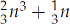. (The thirds are there, as I suspected, although that was far more luck than intuition.) Even with this expression I still can't imagine a physical restacking of cubes (or fractions of cubes) that would lead a student to this result, nor would knowledge of square, rectangular, and triangular numbers.

I find these five patterns intriguing for a number of reasons. First, we go from very basic to very difficult in a quick but logical way. The skeleton tower is a good choice because it incorporates the triangular and rectangular patterns in its solution, and it adds another dimension (literally and figuratively) that suggests new problem-solving approaches, including 3-dimensional spatial reasoning. As for my pyramid-like tower, I like that it re-establishes a disequilibrium for students (and myself). For Algebra 1 students that aren't ready for quadratic regression, being exposed to such a complex pattern might provide some motivation and could be revisited either later in the course or in Algebra 2. It might also be an example I give to students for a "design-your-own" pattern workshop, where students manipulate different permutations of the square, rectangular, and triangular patterns in 2-dimensional and 3-dimensional shapes.

UPDATE (2010.07.02): With great thanks to two friends/followers, I have two more methods of finding the generalization for my pyramidial stack of blocks.

@msmathaddict sent a tweet a link to the Dr. Math portion of the Math Forum that filled in my missing step from my pattern of differences. Now that I've seen the solution it seems obvious, but we can use a system of equations to devise the correct degree equation we're looking for.

My third differences reached a constant, so I knew the equation describing the equation would be third degree. Third degree (cubic) equations are generalized in this form:

From my original figures and table I had four knownpairs: (1,1), (2,6), (3,19), and (4,44). Substituting into the generic cubic form, I can create a system of four equations with four variables:

Simplified, the system becomes:

The system might be most easily solved using matrices (because they are easily entered and manipulated in most graphing calculators):

Solving for, we get, so the general cubic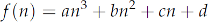becomes.

The second method for deriving the forumla for my pyramidial stack of blocks was sent to me by my friend Andrew Drenner. He opted to use a summation property to summarize the recursive nature of adding increasingly larger layers. He explains:

In a given horizontal slice of the pyramid there iscubes of height. Thus, the problem of finding the total blocks () in a pyramidtall becomes

Given that the summation sequence for squares iscan be expressed as follows: